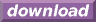#Calcute: freeware calculator

## Sine (sin)

The sine of an angle is defined using the right-triangle formed by this angle: it is the ratio of the triangle's opposite side to its hypotenuse. Calcute implements it with the sin function.

```sin(1)
0.841470984808
```

In the above example, the given angle is in radians.

The triangle involved in the definition of the sine function is commonly shown in the cartesian plane with its adjacent side on the X axis and its angle point located at the origin (0,0). The other end of the triangle's hypotenuse marks a point on the periphery of a circle centered at the origin; the circle's radius is the length of the hypotenuse. If the angle is increased from 0 to 2 pi radians (0 to 360 degrees) then the end point of the hypotenuse traces a full circle. As the angle of the hypotenuse changes, so does its sine value as the shape of the corresponding triangle changes to match.

The sine value remains within the close range [-1, +1].

The sine function is periodic. It is defined for all real numbers. As angle values increase (or decrease) outside the range of [0, 2 pi] radians that represent one full rotation, the value of sin for these angles repeats itself periodically with each new rotation.